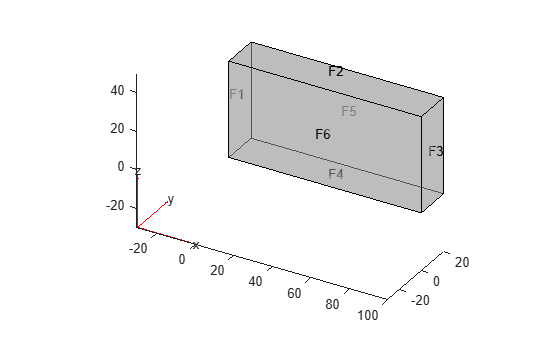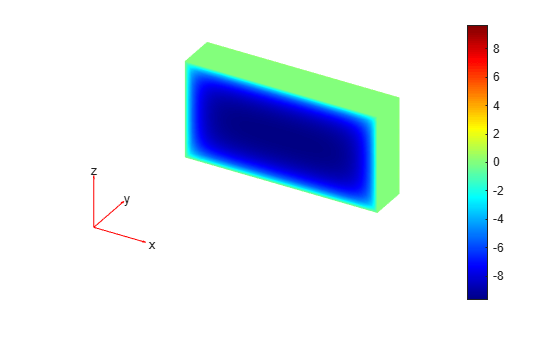# applyBoundaryCondition

Add boundary condition to `PDEModel` container

## Syntax

``applyBoundaryCondition(model,"dirichlet",RegionType,RegionID,Name,Value)``
``applyBoundaryCondition(model,"neumann",RegionType,RegionID,Name,Value)``
``applyBoundaryCondition(model,"mixed",RegionType,RegionID,Name,Value)``
``bc = applyBoundaryCondition(___)``

## Description

example

````applyBoundaryCondition(model,"dirichlet",RegionType,RegionID,Name,Value)` adds a Dirichlet boundary condition to `model`. The boundary condition applies to boundary regions of type `RegionType` with ID numbers in `RegionID`, and with arguments `r`, `h`, `u`, `EquationIndex` specified in the `Name,Value` pairs. For Dirichlet boundary conditions, specify either both arguments `r` and `h`, or the argument `u`. When specifying `u`, you can also use `EquationIndex`.```

example

````applyBoundaryCondition(model,"neumann",RegionType,RegionID,Name,Value)` adds a Neumann boundary condition to `model`. The boundary condition applies to boundary regions of type `RegionType` with ID numbers in `RegionID`, and with values `g` and `q` specified in the `Name,Value` pairs.```

example

````applyBoundaryCondition(model,"mixed",RegionType,RegionID,Name,Value)` adds an individual boundary condition for each equation in a system of PDEs. The boundary condition applies to boundary regions of type `RegionType` with ID numbers in `RegionID`, and with values specified in the `Name,Value` pairs. For mixed boundary conditions, you can use `Name,Value` pairs from both Dirichlet and Neumann boundary conditions as needed.```
````bc = applyBoundaryCondition(___)` returns the boundary condition object.```

## Examples

collapse all

Create a PDE model and geometry.

```model = createpde(1); R1 = [3,4,-1,1,1,-1,-.4,-.4,.4,.4]'; g = decsg(R1); geometryFromEdges(model,g);```

View the edge labels.

```pdegplot(model,"EdgeLabels","on") xlim([-1.2,1.2]) axis equal```Apply zero Dirichlet condition on the edge 1.

```applyBoundaryCondition(model,"dirichlet", ... "Edge",1,"u",0);```

On other edges, apply Dirichlet condition `h*u = r`, where `h = 1` and `r = 1`.

```applyBoundaryCondition(model,"dirichlet", ... "Edge",2:4, ... "r",1,"h",1);```

Create a PDE model and geometry.

```model = createpde(2); R1 = [3,4,-1,1,1,-1,-.4,-.4,.4,.4]'; g = decsg(R1); geometryFromEdges(model,g);```

View the edge labels.

```pdegplot(model,"EdgeLabels","on") xlim([-1.2,1.2]) axis equal```Apply the following Neumann boundary conditions on the edge 4.

```applyBoundaryCondition(model,"neumann", ... "Edge",4, ... "g",[0;.123], ... "q",[0;0;0;0]);```

Apply both types of boundary conditions to a scalar problem. First, create a PDE model and import a simple block geometry.

```model = createpde; importGeometry(model,"Block.stl");```

View the face labels.

`pdegplot(model,"FaceLabels","on","FaceAlpha",0.5)`Set zero Dirichlet conditions on the narrow faces, which are labeled 1 through 4.

```applyBoundaryCondition(model,"dirichlet", ... "Face",1:4,"u",0);```

Set Neumann boundary conditions with opposite signs on faces 5 and 6.

```applyBoundaryCondition(model,"neumann", ... "Face",5,"g",1); applyBoundaryCondition(model,"neumann", ... "Face",6,"g",-1);```

Solve an elliptic PDE with these boundary conditions, and plot the result.

```specifyCoefficients(model,"m",0,"d",0,"c",1,"a",0,"f",0); generateMesh(model); results = solvepde(model); u = results.NodalSolution; pdeplot3D(model,"ColorMapData",u)```Create a PDE model and import a simple block geometry.

```model = createpde(3); importGeometry(model,"Block.stl");```

View the face labels.

`pdegplot(model,"FaceLabels","on","FaceAlpha",0.5)`Set zero Dirichlet conditions on faces 1 and 2.

```applyBoundaryCondition(model,"dirichlet", ... "Face",1:2,"u",[0,0,0]);```

Set Neumann boundary conditions with opposite signs on faces 4, 5, and 6.

```applyBoundaryCondition(model,"neumann", ... "Face",4:5,"g",[1;1;1]); applyBoundaryCondition(model,"neumann", ... "Face",6,"g",[-1;-1;-1]);```

For face 3, apply generalized Neumann boundary condition for the first equation and Dirichlet boundary conditions for the second and third equations.

```h = [0 0 0;0 1 0;0 0 1]; r = [0;3;3]; q = [1 0 0;0 0 0;0 0 0]; g = [10;0;0]; applyBoundaryCondition(model,"mixed","Face",3, ... "h",h,"r",r,"g",g,"q",q);```

Solve an elliptic PDE with these boundary conditions, and plot the result.

```specifyCoefficients(model,"m",0,"d",0,"c",1, ... "a",0,"f",[0;0;0]); generateMesh(model); results = solvepde(model); u = results.NodalSolution; pdeplot3D(model,"ColorMapData",u(:,1))```## Input Arguments

collapse all

PDE model, specified as a `PDEModel` object.

Example: `model = createpde`

Geometric region type, specified as `"Face"` for 3-D geometry or `"Edge"` for 2-D geometry.

Example: `applyBoundaryCondition(model,"dirichlet","Face",3,"u",0)`

Data Types: `char` | `string`

Geometric region ID, specified as a vector of positive integers. Find the region IDs using `pdegplot` with the `"FaceLabels"` (3-D) or `"EdgeLabels"` (2-D) value set to `"on"`.

Example: `applyBoundaryCondition(model,"dirichlet","Face",3:6,"u",0)`

Data Types: `double`

### Name-Value Arguments

Example: `applyBoundaryCondition(model,"dirichlet","Face",1:4,"u",0)`

Dirichlet condition `h*u = r`, specified as a vector with N elements or a function handle. N is the number of PDEs in the system. For the syntax of the function handle form of `r`, see Nonconstant Boundary Conditions.

Example: `"r",[0;4;-1]`

Data Types: `double` | `function_handle`
Complex Number Support: Yes

Dirichlet condition `h*u = r`, specified as an N-by-N matrix, a vector with N^2 elements, or a function handle. N is the number of PDEs in the system. For the syntax of the function handle form of `h`, see Nonconstant Boundary Conditions.

Example: `"h",[2,1;1,2]`

Data Types: `double` | `function_handle`
Complex Number Support: Yes

Generalized Neumann condition `n·(c×``u) + qu = g`, specified as a vector with N elements or a function handle. N is the number of PDEs in the system. For scalar PDEs, the generalized Neumann condition is `n·(c````u) + qu = g```. For the syntax of the function handle form of `g`, see Nonconstant Boundary Conditions.

Example: `"g",[3;2;-1]`

Data Types: `double` | `function_handle`
Complex Number Support: Yes

Generalized Neumann condition `n·(c×``u) + qu = g`, specified as an N-by-N matrix, a vector with N`^2` elements, or a function handle. N is the number of PDEs in the system. For the syntax of the function handle form of `q`, see Nonconstant Boundary Conditions.

Example: `"q",eye(3)`

Data Types: `double` | `function_handle`
Complex Number Support: Yes

Dirichlet conditions, specified as a vector of up to N elements or as a function handle. If `u` has less than N elements, then you must also use `EquationIndex`. The `u` and `EquationIndex` arguments must have the same length. If `u` has N elements, then specifying `EquationIndex` is optional.

For the syntax of the function handle form of `u`, see Nonconstant Boundary Conditions.

Example: `applyBoundaryCondition(model,"dirichlet","Face",[2,4,11],"u",0)`

Data Types: `double`
Complex Number Support: Yes

Index of the known `u` components, specified as a vector of integers with entries from `1` to N. `EquationIndex` and `u` must have the same length.

When using `EquationIndex` to specify Dirichlet boundary conditions for a subset of components, use the `mixed` argument instead of `dirichlet`. The remaining components satisfy the default Neumann boundary condition with the zero values for `"g"` and `"q"`.

Example: `applyBoundaryCondition(model,"mixed","Face",[2,4,11],"u",[3,-1],"EquationIndex",[2,3])`

Data Types: `double`

Vectorized function evaluation, specified as `"on"` or `"off"`. This evaluation applies when you pass a function handle as an argument. To save time in function handle evaluation, specify `"on"`, assuming that your function handle computes in a vectorized fashion. See Vectorization. For details of this evaluation, see Nonconstant Boundary Conditions.

Example: `applyBoundaryCondition(model,"dirichlet","Face",[2,4,11],"u",@ucalculator,"Vectorized","on")`

Data Types: `char` | `string`

## Output Arguments

collapse all

Boundary condition, returned as a BoundaryCondition Properties object. The `model` object contains a vector of `BoundaryCondition` objects. `bc` is the last element of this vector.

## Tips

• When there are multiple boundary condition assignments to the same geometric region, the toolbox uses the last applied setting.

• To avoid assigning boundary conditions to a wrong region, ensure that you are using the correct geometric region IDs by plotting and visually inspecting the geometry.

• If you do not specify a boundary condition for an edge or face, the default is the Neumann boundary condition with the zero values for `"g"` and `"q"`.

## Version History

Introduced in R2015a